# Ncert Class 6 Math Fractions Exercise 7.6

Fractions Class 6 Ex. 7.6

New Ncert Class 6, Chapter 7 Math Free Solution.

Exercise 7.6

Question 1 :- Solve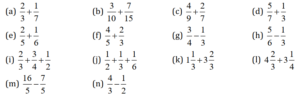Solution 1:-

(a) Since, L.C.M of 7 and 3 is 7×3 = 21;

2/3 + 1/7 = [(2×7)+(1×3)]/(3×7) = (14+3)/21 = 17/21;

(b) Since, L.C.M of 10 and 15 is 10×15 = 150;

3/10 + 7/15 = [(3×15)+(7×10)]/(10×15) = (45+70)/150 = 115/150 = 23/30;

(c) Since, L.C.M of 9 and 7 is 9×7 = 63;

4/9 + 2/7 =[(4×7)+(2×9)]/(9×7) = (28+18)/63 = 46/63;

(d) Since, L.C.M of 7 and 3 is 7×3 = 21;

5/7 +1/3 = [(5×3)+(1×7)]/(3×7) = (15+7)/21 = 22/21;

(e) Since, L.C.M of 5 and 6 is 5×6 = 30;

2/5 +1/6 = [(2×6)+(1×5)]/(5×6) = (12+5)/30 = 17/30;

(f) Since, L.C.M of 5 and 3 is 5×3 = 15;

4/5 + 2/3 = [(4×3)+(2×5)]/(5×3) = (12+10)/15 = 22/15;

(g) Since, L.C.M of 4 and 3 is 4×3 = 12;

3/4 – 1/3 = [(3×3)-(1×4)]/(4×3) = (9-4)/12 = 5/12;

(h) Since, L.C.M of 6 and 3 is 6×3 = 18;

5/6 – 1/3 = [(5×3)-(1×6)]/(6×3) = (15-6)/18 = 9/18 = 1/2;

(i) Since, L.C.M of 4 and 2 is 4×2 = 8;
2/3 + 3/4 + 1/2 =  2/3 + [(3×2)+(1×4)]/(2×4) = 2/3 + [(6+4)/8] = 2/3 + 10/8;

Now, for 2/3 + 10/8

L.C.M of 3 and 8 is 3×8 = 24;
2/3 + 10/8 = (2×8)+(3×10)/(3×8) = (16+30)/24 = 46/24 = 23/12;

(j) Since, L.C.M of 3 and 6 is 3×6 = 18;
1/2 + 1/3 + 1/6 =  1/2 + [(1×6)+(1×3)]/(3×6) = 1/2 + [(6+3)/18] = 1/2 + 9/18 = 1/2 + 1/2;

Now, for 1/2 + 1/2;

L.C.M of 1/2 and 1/2 is 2;
1/2 + 1/2 = (1+1)+/2 = 2/2 = 1;

(k)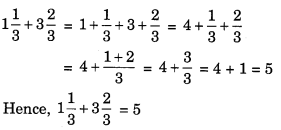(l)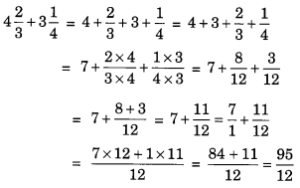(m) Since, L.C.M of 16/5 and 7/5 is 5;

16/5 – 7/5 = (16-7)/5 = 9/5;

(n) Since, L.C.M of 4/3 and 1/2 is 3×2 = 6;

4/3 – 1/2 = [(4×2)-(1×3)]/(3×2) = (8-3)/6 = 5/6;

Question 2 :- Sarita bought 2/5 metre of ribbon and Lalita 3/4 metre of ribbon. What is the total length of the ribbon they bought?

Solution 2:-
Sarita’s purchase of a length of ribbon = 2/5 metre
Lalita’s purchase of a length of ribbon = 3/4 metre
So, the length of ribbon purchase by Sarita and Lalita is

2/5 + 3/4 = [(2×4)+(5×3)]/(5×4) = (8+15)/20 = 23/20;

Hence, the required length = 23/20 metre

Question 3 :- Naina was given 1,1/2 piece of cake and Najma was given 1,1/3 piece of cake. Find total-amount of cake was given to both of them.

Solution 3:-
Naina receives a piece of cake = 1,1/2
Najma receives a piece of cake = 1,1/3
The piece of cake given to both Naina and Najma is (1,1/2) + (1,1/3);

1+ 1/2 + 1 + 1/3 = 2 + 1/2 + 1/3 = 2 + [(1×3)+(1×2)]/(2×3) = 2+ (3+2)/6 = 2+ 5/6 = 2,5/6;

[(2×6)+(1×5)]/6 = (12+5)/6 = 17/6

So, as a result the total amount of cake given to both of them are  = 2,5/6.

Question 4 :- Fill in the boxes:Solution 4:-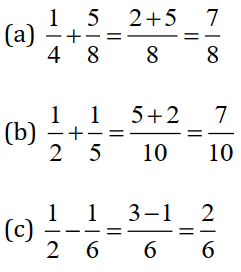2/6 = 1/3;

Question 5 :- Complete the addition-subtraction box.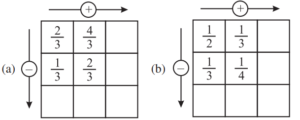Solution 5:-

(a)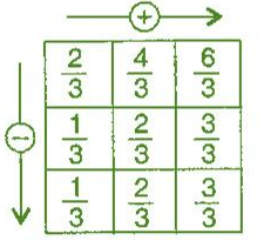(b)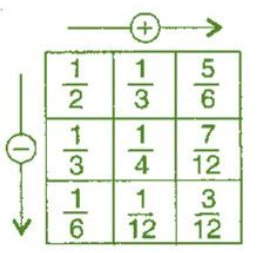Question 6 :- A piece of wire 7/8 metre long broke into two-piece. One piece was 1/4 metre long. How long is the other piece?

Solution 6:-
The total length of the wire is  = 7/8 metre
The length of one piece of wire is = 1/4 metre;

So,  Length of the other piece = 7/8 – 1/4
L.C.M of 8 and 4 = 32

7/8 – 1/4 = [(7×4)-(1×8)]/(4×8) = (28-8)/32 = 20/32 = 5/8;

Therefore, the length of the other piece = 5/8 metre.

Question 7 :- Nandini’s house is 9/10 km from her-school. She walked some-distance and then took a bus for 1/2km to reach-school. How far did she walk?

Solution 7:-
The total distance from house to school is = 9/10 km.
The distance travelled by Nandini by bus is = 1/2 km
The distance travelled by her on foot is =  9/10 – 1/2

Since L.C.M of 10 and 2 is 20;

9/10 – 1/2 = [(9×2)-(1×10)]/(10×2) = (18-10)/20 = 8/20 = 2/5

So, therefore the distance travelled by her on-foot is = 2/5km.

Question 8 :- Asha and Samuel have bookshelves of the same-size partly filled with book. Asha’s shelf is 5/6th full and Samuel’s shelf is 2/5th full. Whose bookshelf is more full? By what fraction?

Solution 8:-
Asha’s shelf is 5/6th full
and Samuel’s shelf is 2/5th full
Comparing 5/6 and 2/5
Since, L.C.M of 6 and 5 = 30

5/6 = (5×5)/(6×5) = 25/30 and

2/5 = (2×6)/(5×6) = 12/30

So, as a result, Asha’s shelf is full more than Samuel’s shelf.

{25/30} – {12/30} = (25-12)/30 = 13/30;

So, as a result, (13/30)th fraction is more full of Asha’s shelf.

Question 9 :- Jaidev takes 2,1/5 minutes to walk across the school ground. Rahul takes 7/4 minutes to do the same. Who takes less time and by what fraction?

Solution 9:-
Jaidev takes 2,1/5 minutes = 2+ (1/5) = [(2×5)+(1×1)]/ 5 = (10+1)/5 = 11/5 minutes;
Rahul takes 7/4 minutes
Comparing 2,1/5 minutes and 7/4 minutes

Since the L.C.M 11/5 and 7/4 is 20
11/5 = (11×4)/5×4 = 44/20;
7/4 = (7×5)/(4×5) = 35/20;

Rahul therefore needs less time than Jaidev to travel the same distance.

The diffrence 11/5 and 7/4 is
11/5 – 7/4 = [(11×4) – (7×5)]/20 =(44-35)/20 = 9/20;

Therefore, Rahul takes 9/20 minutes less to across the school ground.